# Cartesian product

"Cartesian square" redirects here. For Cartesian squares in category theory, see Cartesian square (category theory).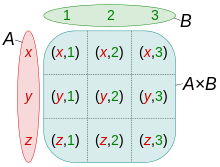Cartesian productof the setsandIn mathematics, a Cartesian product is a mathematical operation that returns a set (or product set or simply product) from multiple sets. That is, for sets A and B, the Cartesian product A × B is the set of all ordered pairs (a, b) where aA and bB. Products can be specified using set-builder notation, e.g.A table can be created by taking the Cartesian product of a set of rows and a set of columns. If the Cartesian product rows × columns is taken, the cells of the table contain ordered pairs of the form (row value, column value).

More generally, a Cartesian product of n sets, also known as an n-fold Cartesian product, can be represented by an array of n dimensions, where each element is an n-tuple. An ordered pair is a 2-tuple or couple.

The Cartesian product is named after René Descartes, whose formulation of analytic geometry gave rise to the concept, which is further generalized in terms of direct product.

## Examples

### A deck of cards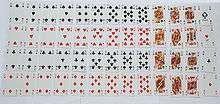Standard 52-card deck

An illustrative example is the standard 52-card deck. The standard playing card ranks {A, K, Q, J, 10, 9, 8, 7, 6, 5, 4, 3, 2} form a 13-element set. The card suits {♠, , , ♣} form a four-element set. The Cartesian product of these sets returns a 52-element set consisting of 52 ordered pairs, which correspond to all 52 possible playing cards.

Ranks × Suits returns a set of the form {(A, ♠), (A, ), (A, ), (A, ♣), (K, ♠), ..., (3, ♣), (2, ♠), (2, ), (2, ), (2, ♣)}.

Suits × Ranks returns a set of the form {(♠, A), (♠, K), (♠, Q), (♠, J), (♠, 10), ..., (♣, 6), (♣, 5), (♣, 4), (♣, 3), (♣, 2)}.

Both sets are distinct, even disjoint.

### A two-dimensional coordinate system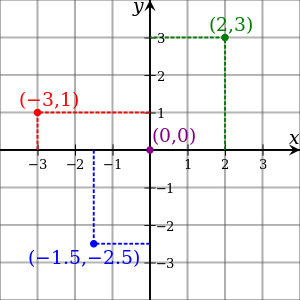Cartesian coordinates of example points

The main historical example is the Cartesian plane in analytic geometry. In order to represent geometrical shapes in a numerical way and extract numerical information from shapes' numerical representations, René Descartes assigned to each point in the plane a pair of real numbers, called its coordinates. Usually, such a pair's first and second components are called its x and y coordinates, respectively; cf. picture. The set of all such pairs (i.e. the Cartesian product ℝ×ℝ with ℝ denoting the real numbers) is thus assigned to the set of all points in the plane.

## Most common implementation (set theory)

A formal definition of the Cartesian product from set-theoretical principles follows from a definition of ordered pair. The most common definition of ordered pairs, the Kuratowski definition, is. Note that, under this definition,, whererepresents the power set. Therefore, the existence of the Cartesian product of any two sets in ZFC follows from the axioms of pairing, union, power set, and specification. Since functions are usually defined as a special case of relations, and relations are usually defined as subsets of the Cartesian product, the definition of the two-set Cartesian product is necessarily prior to most other definitions.

### Non-commutativity and non-associativity

Let A, B, C, and D be sets.

The Cartesian product A × B is not commutative,because the ordered pairs are reversed except if at least one of the following conditions is satisfied:

• A is equal to B, or
• A or B is the empty set.

For example:

A = {1,2}; B = {3,4}
A × B = {1,2} × {3,4} = {(1,3), (1,4), (2,3), (2,4)}
B × A = {3,4} × {1,2} = {(3,1), (3,2), (4,1), (4,2)}
A = B = {1,2}
A × B = B × A = {1,2} × {1,2} = {(1,1), (1,2), (2,1), (2,2)}
A = {1,2}; B = ∅
A × B = {1,2} × ∅ = ∅
B × A = ∅ × {1,2} = ∅

Strictly speaking, the Cartesian product is not associative (unless one of the involved sets is empty).If for example A = {1}, then (A × A) × A = { ((1,1),1) } ≠ { (1,(1,1)) } = A × (A × A).

### Intersections, unions, and subsetsExample sets A={y∈ℝ:1≤y≤4}, B={x∈ℝ:2≤x≤5}, and C={x∈ℝ:4≤x≤7}, demonstrating A×(B∩C)=(A×B)∩(A×C), A×(B∪C)=(A×B)∪(A×C), and A×(B\C)=(A×B)\(A×C).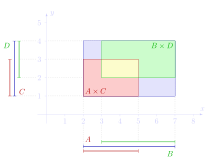(A∪B)×(C∪D)≠(A×C)∪(B×D) can be seen from the same example.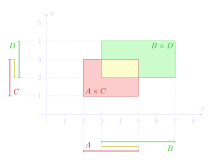Example sets A={x∈ℝ:2≤x≤5}, B={x∈ℝ:3≤x≤7}, C={y∈ℝ:1≤y≤3}, and D={y∈ℝ:2≤y≤4}, demonstrating (A∩B)×(C∩D)=(A×C)∩(B×D).

The Cartesian product behaves nicely with respect to intersections, cf. left picture.In most cases the above statement is not true if we replace intersection with union, cf. middle picture.In fact, we have that:For the set difference we also have the following identity:Here are some rules demonstrating distributivity with other operators (cf. right picture):wheredenotes the absolute complement of A.

Other properties related with subsets are:### Cardinality

The cardinality of a set is the number of elements of the set. For example, defining two sets: A = {a, b} and B = {5, 6}. Both set A and set B consist of two elements each. Their Cartesian product, written as A × B, results in a new set which has the following elements:

A × B = {(a,5), (a,6), (b,5), (b,6)}.

Each element of A is paired with each element of B. Each pair makes up one element of the output set. The number of values in each element of the resulting set is equal to the number of sets whose cartesian product is being taken; 2 in this case. The cardinality of the output set is equal to the product of the cardinalities of all the input sets. That is,

|A × B| = |A| · |B|.

Similarly

|A × B × C| = |A| · |B| · |C|

and so on.

The set A × B is infinite if either A or B is infinite and the other set is not the empty set.

## n-ary product

### Cartesian power

The Cartesian square (or binary Cartesian product) of a set X is the Cartesian product X2 = X × X. An example is the 2-dimensional plane R2 = R × R where R is the set of real numbers: R2 is the set of all points (x,y) where x and y are real numbers (see the Cartesian coordinate system).

The cartesian power of a set X can be defined as:An example of this is R3 = R × R × R, with R again the set of real numbers, and more generally Rn.

The n-ary cartesian power of a set X is isomorphic to the space of functions from an n-element set to X. As a special case, the 0-ary cartesian power of X may be taken to be a singleton set, corresponding to the empty function with codomain X.

### Finite n-ary product

The Cartesian product can be generalized to the n-ary Cartesian product over n sets X1, ..., Xn:It is a set of n-tuples. If tuples are defined as nested ordered pairs, it can be identified to (X1 × ... × Xn−1) × Xn.

### Infinite products

It is possible to define the Cartesian product of an arbitrary (possibly infinite) indexed family of sets. If I is any index set, andis a family of sets indexed by I, then the Cartesian product of the sets in X is defined to bethat is, the set of all functions defined on the index set such that the value of the function at a particular index i is an element of Xi. Even if each of the Xi is nonempty, the Cartesian product may be empty if the axiom of choice (which is equivalent to the statement that every such product is nonempty) is not assumed.

For each j in I, the functiondefined byis called the jth projection map.

An important case is when the index set is, the natural numbers: this Cartesian product is the set of all infinite sequences with the ith term in its corresponding set Xi. For example, each element ofcan be visualized as a vector with countably infinite real number components. This set is frequently denoted, or.

The special case Cartesian exponentiation occurs when all the factors Xi involved in the product are the same set X. In this case,is the set of all functions from I to X, and is frequently denoted XI. This case is important in the study of cardinal exponentiation.

The definition of finite Cartesian products can be seen as a special case of the definition for infinite products. In this interpretation, an n-tuple can be viewed as a function on {1, 2, ..., n} that takes its value at i to be the ith element of the tuple (in some settings, this is taken as the very definition of an n-tuple).

## Other forms

### Abbreviated form

If several sets are being multiplied together, e.g. X1, X2, X3, …, then some authors choose to abbreviate the Cartesian product as simply ×Xi.

### Cartesian product of functions

If f is a function from A to B and g is a function from X to Y, their Cartesian product f × g is a function from A × X to B × Y withThis can be extended to tuples and infinite collections of functions. Note that this is different from the standard cartesian product of functions considered as sets.

## Definitions outside of set theory

### Category theory

Although the Cartesian product is traditionally applied to sets, category theory provides a more general interpretation of the product of mathematical structures. This is distinct from, although related to, the notion of a Cartesian square in category theory, which is a generalization of the fiber product.

Exponentiation is the right adjoint of the Cartesian product; thus any category with a Cartesian product (and a final object) is a Cartesian closed category.

### Graph theory

In graph theory the Cartesian product of two graphs G and H is the graph denoted by G × H whose vertex set is the (ordinary) Cartesian product V(G) × V(H) and such that two vertices (u,v) and (u′,v′) are adjacent in G × H if and only if u = u and v is adjacent with v′ in H, or v = v and u is adjacent with u′ in G. The Cartesian product of graphs is not a product in the sense of category theory. Instead, the categorical product is known as the tensor product of graphs.